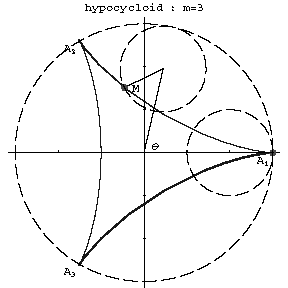Hypocycloid

A plane curve which is the trajectory of a point on a circle rolling along a second circle while osculating it from inside. The parametric equations are

$$x(\theta)=(R-r)\cos\theta+r\cos\left[(R-r)\frac\theta r\right],$$

$$y(\theta)=(R-r)\sin\theta-r\sin\left[(R-r)\frac\theta r\right],$$

where $r$ is the radius of the moving circle, $R$ is the radius of the fixed circle and $\theta$ is the angle between the radius vector of the centre of the moving circle with the $x$-axis (assuming the trajectory passes through $(0,R)$). Depending on the size of the modulus $m=R/r$, hypocycloids of different forms are obtained. If $m$ is an integer, the curve consists of $m$ non-intersecting branches (Fig. a). The points of return $A_1,\ldots,A_m$ have polar coordinates $\rho=R$, $\phi=2k\pi/m$, $k=0,\ldots,m-1$. If $m$ is irrational, the number of branches is infinite, and the point $M$ does not return to its initial location; if $m$ is rational, the hypocycloid is a closed algebraic curve. The arc length from the point $\theta=0$ is

$$l=\frac{8R(m-1)}{m^2}\sin^2\frac\theta4.$$

The radius of the curvature is

$$r_k=\frac{4R(m-1)}{m^2(m-2)}\sin\frac\theta2.$$

$m=3$.Figure: h048530a

If the point is not located on the rolling circle, but outside (or inside) it, the curve is said to be a lengthened (shortened) hypocycloid, or hypotrochoid. If $m=2$ the hypocycloid is a segment of a straight line; if $m=3$, it is a Steiner curve; if $m=4$, it is an astroid. Hypocycloids belong to the so-called cycloidal curves.

How to Cite This Entry:
Hypocycloid. Encyclopedia of Mathematics. URL: http://encyclopediaofmath.org/index.php?title=Hypocycloid&oldid=42491
This article was adapted from an original article by D.D. Sokolov (originator), which appeared in Encyclopedia of Mathematics - ISBN 1402006098. See original article